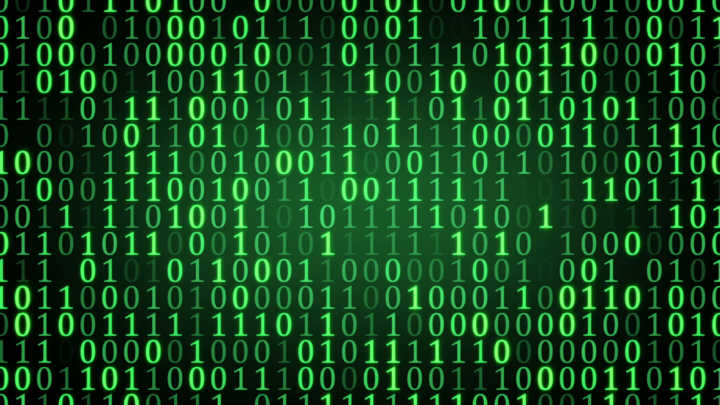# Ones and Zeros

## What is the smallest number evenly divisible by 225 that contains only the digits 1 and 0?I like puzzles that are easy to state and don’t require a lengthy explanation. Today’s puzzle falls into that category. It comes from my good friend of 30 years, Ken O’Brien, who asked me this simple but perplexing question: “What is the smallest number evenly divisible by 225 that contains only the digits 1 and 0?”

If you’re not able to find the answer analytically, see if you can solve it algorithmically. In other words, see if you can come up with a procedure (brute force method or something more efficient) for finding the answer and leave me a comment with your results (I’ll post the answer on Tuesday). Oh, and by the way, don’t try to use ones and zeros for commercial purposes – they’re patented.

Solution: I know of three ways to solve this problem:

The Slow Search Method – This approach starts with 225 and multiplies it by an ever-increasing sequence of integers looking for a number that contains only ones and zeros. Here’s the Python code to implement this method: import time

start = 225 # starting number num = start cnt = 1 binary_digits = (‘0’, ‘1’) keep_looking = True

# capture start time

start_time = time.clock()

while keep_looking: num += start # check for all 1s and 0s in num keep_looking = False # assume we found desired number for i in str(num): if i not in binary_digits: keep_looking = True # nope, not the desired number break cnt += 1

elapsed = time.clock() - start_time # calculate elapsed time

# we exit the above loop when we’ve found the desired number

print(‘after’, cnt, ‘iterations and’, elapsed, ‘seconds:‘, num) Which prints the following result: after 49382716 iterations and 83.33 seconds: 11111111100

The Fast Search Method – This strategy observes that the desired result looks like a binary number (albeit in base 10) so it tests a sequence of binary numbers, treating each as a base 10 number, looking for one that’s evenly divisible by 225. This is much faster than the previous method because it automatically skips all the base 10 numbers that have digits other than 1 and 0. Here’s the Python code: import time

def convert(num, b1, b2): “‘convert the passed num from base b1 to base b2”’ result = 0 digits = [] while num: digits.append(num % b1)
num //= b1 digits.reverse() for i in digits: result = (result * b2) + i return result

start = 225 num = 1000 # start with smallest possible answer > 225 cnt = 1

# capture start time

start_time = time.clock()

while True: if (num % start) == 0:
break # convert to base 2, increment, then convert back to base 10 num = convert(num, 10, 2) num += 1 num = convert(num, 2, 10) cnt += 1

elapsed = time.clock() - start_time # calculate elapsed time

# we exit the above loop when we’ve found the desired number

print(‘after’, cnt, ‘iterations and’, elapsed, ‘seconds:‘, num) which prints the following result: after 2037 iterations and 0.04 seconds: 11111111100

The Analytical Method – Because 225 ends in 25, multiples of 225 will end in one of four possible digit pairs: 25, 50, 75 or 00. The only one that meets our requirements (only 1s and 0s allowed) is the last one so we know that the result must end with two 0s. We can also see that 225 is divisible by 9 (recall the rule from grade school about summing the digits to check if a number is divisible by 9) and, therefore, any multiple of 225 must also be divisible by 9. Thus, the digits in the result must also sum to 9, so the smallest possible number meeting our requirements will contain nine consecutive 1s and will end with two 0s: 11111111100. Obviously the fast search method is much more efficient than the slow search method (nearly 50,000,000 fewer loop iterations and 2,000 times faster) but the analytical approach is the clear winner because it doesn’t require any searching at all. The most efficient program of all is the one you don’t need to write. :)